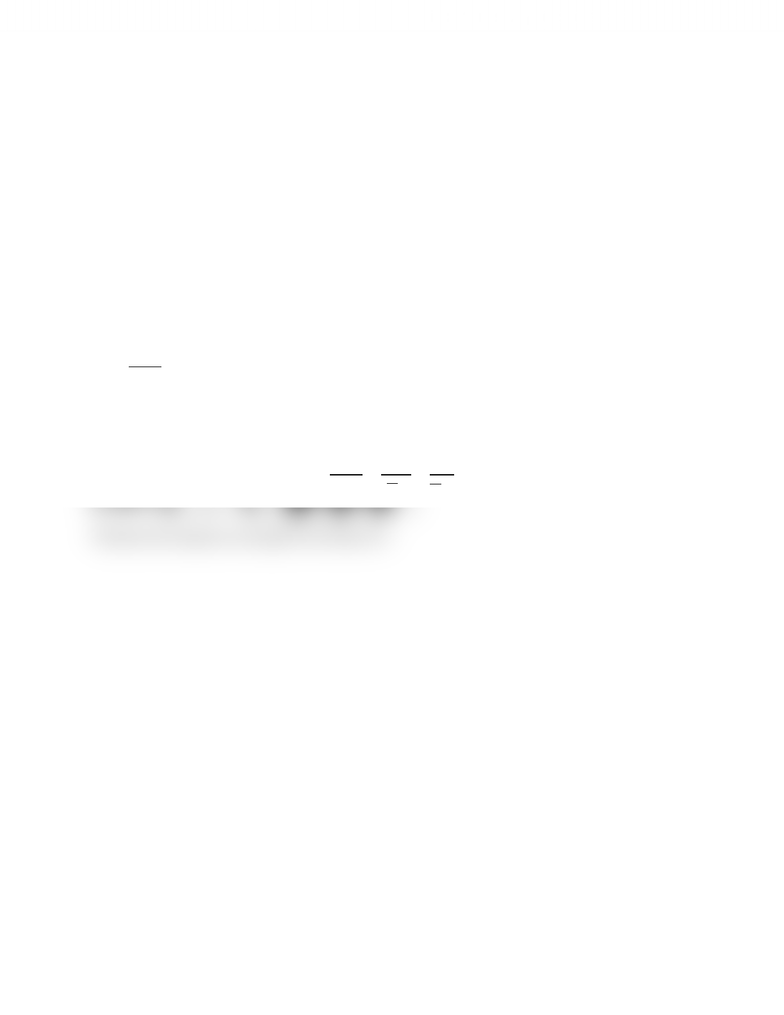Class Notes (1,100,000)
CA (630,000)
UTSG (50,000)
MAT (4,000)
MAT136H1 (900)
all (200)
Lecture

# 11.1 Sequences Question #3 (Medium)

Department
Mathematics
Course Code
MAT136H1
Professor
all

This preview shows half of the first page. to view the full 1 pages of the document.11.1 Infinite Sequences & Series
Infinite Sequences
Question #3 (Medium): Convergent Sequence and Finding Its Limit
Strategy
The long and tedious way would be to plug in each n number for the terms in the sequence and observe
if the pattern is convergent or divergent. This may not always be correct. Much better approach is to
determine the convergence and divergence systematically by treating the n as a variable, simplifying and
applying limit to it as   
Sample Question
Determine if the sequence converges or diverges. If it converges, find the limit.


Solution
Treating the n as a variable, the general sequence formula can be simplified. Plugging in   is of no
use at this point as both numerator and denominator will approach .
Divide top and bottom by then: 



As   :   


  
Therefore the sequence converges to the value of .